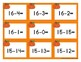# Pumpkin Themed Hands On Subtraction CenterSubject
Resource Type
File Type

PDF

(315 KB|32 pages)
Product Rating
Standards
Also included in:
1. These themed subtraction centers are hands on centers for students to practice subtracting numbers within 20. All subtraction facts from 20-20 down to 0-0 are included in this packet.What is included?A math mat for students to use manipulatives to solve math problemsA set of pumpkin themed math card
\$15.00
\$10.00
Save \$5.00
• Product Description
• StandardsNEW

This pumpkin themed subtraction center is a hands on center for students to practice subtracting numbers within 20. All subtraction facts from 20-20 down to 0-0 are included in this packet.

What is included?

• A math mat for students to use manipulatives to solve math problems
• A set of pumpkin themed math cards that included all of the subtraction facts 20-20 down to 0-0.
• Two different recording options. One full page option and one page with recording sheets for up to 3 students.
Add and subtract within 20, demonstrating fluency for addition and subtraction within 10. Use strategies such as counting on; making ten (e.g., 8 + 6 = 8 + 2 + 4 = 10 + 4 = 14); decomposing a number leading to a ten (e.g., 13 - 4 = 13 - 3 - 1 = 10 - 1 = 9); using the relationship between addition and subtraction (e.g., knowing that 8 + 4 = 12, one knows 12 - 8 = 4); and creating equivalent but easier or known sums (e.g., adding 6 + 7 by creating the known equivalent 6 + 6 + 1 = 12 + 1 = 13).
Fluently add and subtract within 5.
Solve addition and subtraction word problems, and add and subtract within 10, e.g., by using objects or drawings to represent the problem.
Represent addition and subtraction with objects, fingers, mental images, drawings, sounds (e.g., claps), acting out situations, verbal explanations, expressions, or equations.
Total Pages
32 pages
N/A
Teaching Duration
N/A
Report this Resource to TpT
Reported resources will be reviewed by our team. Report this resource to let us know if this resource violates TpT’s content guidelines.